Software Engineer | Curious Thinker | Life-Long-Learner | https://www.linkedin.com/in/abdulsalamone/

# Solving Tower of Hanoi Puzzle in Python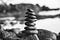Photo by Milada Vigerova on Unsplash

“The Tower of Hanoi is a mathematical game or puzzle. It consists of three rods and a number of disks of different sizes, which can slide onto any rod. The puzzle starts with the disks in a neat stack in ascending order of size on one rod, the smallest at the top, thus making a conical shape.

The objective of the puzzle is to move the entire stack to last rod, obeying the following simple rules:

• Each move consists of taking the upper disk from one of the stacks and placing…

# Dijkstra Algorithm in Python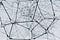Photo by Alina Grubnyak on Unsplash

It is a well-known Algorithm used to find the shortest distance and shortest path from a single source node to all other nodes in a graph. This algorithm only works on graphs, where there are no negative cycles in them (i.e. a cycle whose edges sum to a negative value).

# The algorithm

1. Create a set “seen” to keep track of visited nodes.
2. Create a dictionary “parentsMap” for parents map to reconstruct the path after the execution of the algorithm.
3. Create a dictionary “nodeCosts” for keeping track of minimum costs for reaching different nodes from the source node, and initialize the cost for…

# Typing Effect in React

Today, we will learn how to implement a Typing Effect in React from scratch.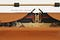Photo by Rafaela Biazi on Unsplash

A working Example can be found here.

# Setting-Up

Start by creating a starter react app using `npx create-react-app my-app` or follow the procedure from here.

Go to App.js, remove all redundant Elements, and add a `h1`tag where the typing effect will take place.

Now import to add additional hooks using `import {useEfect, useState} from "react";`

Create an array of words that will be type one by one.

Now we have to keep track of the index of the word array for typing a specific word, so we…

# All Pythagorean Triplets Less Than Equal to N in Optimal Time Complexity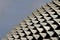Photo by Nick Fewings on Unsplash

Pythagorean Triplets are set of three ordered positive integers (x,y,z) such that

`                       x^2 + y^2 = z^2    --- (1)where,x < y < z <= N`

Generating Pythagorean triplets simply using two loops by iterating over x and y for all possible…

# Mandelbrot Set with Python

“A Mandelbrot set marks the set of points in the complex plane such that the corresponding Julia set is connected and not computable. The Mandelbrot set is the set obtained from the recurrence relation, Z_(n) = Z²_(n-1) + c, where, Z_(0) = c. where c is a complex number” — Wolfram Mathworld.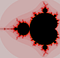Mandelbrot Set

The colors in a Mandelbrot set represents the iterations at which that complex number diverges to infinity (we can use here some threshold instead of infinity). …

# Search Technique on Graphs: Breadth-First Search

## Search far and wide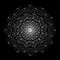Image by AnaSophia Eiseman

Breadth-first search (BFS) is a common search technique on graphs. BFS is a companion of depth-first search (DFS). While DFS traverses the graph depth-wise, BFS does it breadth-wise. It’s used to find a node in a graph. It may also be used to get the path to that node from a given node or to just traverse all the nodes and edges in a graph.

## Why use BFS over DFS?

Let’s say we want to find a node in a tree where we know in advance the tree is not bushy (higher diameter) and the node lies somewhere in the middle of the tree. …

# Search Technique on Graphs: Depth-First Search

## Dive into search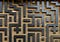Photo by Mitchell Luo on Unsplash

Depth-First Search (DFS) is a common search technique on graphs. It is used to find a node in a graph and may also be used to get the path to that node from a given node or to just traverse all the nodes and edges in a graph.

It can also be augmented to do many more interesting things such as finding connected components, strongly connected components, minimum spanning tree, cycles in graphs, topological sort, etc.

For a refresher and basic concepts on graphs visit my previous post, graph theory algorithms simplified.

All the code used in this series can…

# Graph Theory Algorithms “Simplified”

## Graph theory 101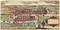The City of Königsberg, Historic Cities Research Project

Graph theory is a very broad branch of mathematics and it is highly applicable in real-world problems. Originally, graph theory was “invented” to solve real-world problems and after that, it was hijacked by abstract mathematicians like all other branches of mathematics.

In this tutorial and subsequent tutorials, we will look at some graph theory algorithms and their implementations in Python. Now, back to the meat of the subject.

# What Is a Graph?

In a nutshell, a graph is a set of vertices/nodes and edges. If you are not comfortable with “set” replace it with collection.